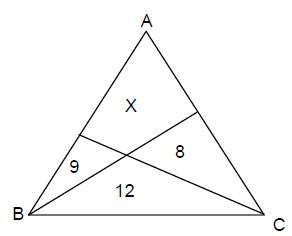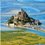# Find the area....Triangle ABC is divided into four parts, and the area of each part is as shown in the figure. Find the value of x, where x is the area.Note by Kshitij Johary
6 years, 8 months ago

This discussion board is a place to discuss our Daily Challenges and the math and science related to those challenges. Explanations are more than just a solution — they should explain the steps and thinking strategies that you used to obtain the solution. Comments should further the discussion of math and science.

When posting on Brilliant:

• Use the emojis to react to an explanation, whether you're congratulating a job well done , or just really confused .
• Ask specific questions about the challenge or the steps in somebody's explanation. Well-posed questions can add a lot to the discussion, but posting "I don't understand!" doesn't help anyone.
• Try to contribute something new to the discussion, whether it is an extension, generalization or other idea related to the challenge.

MarkdownAppears as
*italics* or _italics_ italics
**bold** or __bold__ bold
- bulleted- list
• bulleted
• list
1. numbered2. list
1. numbered
2. list
Note: you must add a full line of space before and after lists for them to show up correctly
paragraph 1paragraph 2

paragraph 1

paragraph 2

[example link](https://brilliant.org)example link
> This is a quote
This is a quote
    # I indented these lines
# 4 spaces, and now they show
# up as a code block.

print "hello world"
# I indented these lines
# 4 spaces, and now they show
# up as a code block.

print "hello world"
MathAppears as
Remember to wrap math in $$ ... $$ or $ ... $ to ensure proper formatting.
2 \times 3 $2 \times 3$
2^{34} $2^{34}$
a_{i-1} $a_{i-1}$
\frac{2}{3} $\frac{2}{3}$
\sqrt{2} $\sqrt{2}$
\sum_{i=1}^3 $\sum_{i=1}^3$
\sin \theta $\sin \theta$
\boxed{123} $\boxed{123}$

Sort by:

Okay, let me give an explanation with slightly less confusing variable names. We use the notation $[X]$ to denote the area of triangle $X$.

Let the point on side $AB$ which is hit by the line emanating from $C$ be $K$. Define $L$ on $AC$ similarly. Let $M$ be the intersection of lines $CK$ and $BL$. Let the areas of $\triangle AKM$ and $\triangle ALM$ be $R$ and $S$ respectively.

Notice that $\frac{[ALM]}{[LMC]} = \frac{[ABL]}{[BLC]} = \frac{AL}{LC}$. In terms of $R$, $S$, and numbers, we get that $\frac{S}8 = \frac{9+R+S}{12+8}, \quad \text{or} \\ \frac{S}{9+R+S} = \frac{8}{12+8}.$ Also, notice that $\frac{[AKM]}{[KMB]} = \frac{[ACK]}{[CKB]} = \frac{AK}{KB}$. In terms of $R$, $S$, and numbers, we get that $\frac{R}{9} = \frac{8+R+S}{9+12}, \quad \text{or} \\ \frac{R}{8+R+S} = \frac{9}{9+12}.$

Finally, we solve. After simplifying and cross-multiplying both equations, we get $5S = 18+2R+2S$ and $7R = 24+3R+3S$. From the second equation, we get that $R = 6+\frac34S$, and substituting in the first equation, $5S = 18 + 12 + \frac32S + 2S$. Hence, $\frac32S = 30$, or $S = 20$. Substituting this back in the first equation gives $42=2R$, or $21=R$.

Hence, the requested area is $20+21 = \boxed{41}.$

- 6 years, 8 months ago

Let the cevians with endpoints $B$ and $C$ intersect $AC$ and $AB$ at $I_\text{swag}$ and $I_\text{yolo}$, respectively. Let $BI_\text{swag}$ and $CI_\text{yolo}$ intersect at $I_\text{sa}$. Let $area(\triangle AI_\text{sa}I_\text{yolo})$ be $A_\text{bad}$ and $area(\triangle AI_\text{sa}I_\text{swag})$ be $A_\text{toed}$. Condsider triangles $\triangle AI_\text{yolo}B$ and $\triangle AI_\text{yolo}I_\text{sa}$. Since they share the same altitude, creary $\frac{A_\text{bad}}{9+A_\text{bad}+A_\text{toed}}=\frac8{8+12}.$ Similarly, consider triangles $\triangle AI_\text{swag}C$ and $\triangle AI_\text{swag}I_\text{sa}$. Since they share an altitude, creary $\frac{A_\text{bad}}{A_\text{bad}+A_\text{toed}+8}=\frac9{9+21}.$ Solving for $A_\text{bad}$ and $A_\text{toed}$ gives $A_\text{bad}=21$ and $A_\text{toed}$, so the answer is $A_\text{bad}+A_\text{toed}={\huge A_\text{BAD TOED}}=41$.

EDIT: why is this getting downvoted? It's perfectly legit.

- 6 years, 8 months ago

u nailed it!

- 6 years, 8 months ago

I think I've learned a little from you. Thank you. :)

- 6 years, 8 months ago

- 6 years, 8 months ago

yolo sa

EDIT: Ok I'll actually elaborate the most confusing part.

So note that triangles $\triangle I_\text{yolo}CI_\text{sa}$ and $\triangle BI_\text{sa}C$ have the same height so the ratio of their areas is the same as the ratio of their bases. Thus $\frac{I_\text{yolo}I_\text{sa}}{I_\text{yolo}I_\text{sa}+I_\text{sa}B}=\frac8{8+12}.$ So that's how I got $\frac{A_\text{bad}}{9+A_\text{bad}+A_\text{toed}}=\frac8{8+12}.$

- 6 years, 8 months ago

In a family of 3children what is the probability of having at least one boy

- 4 years, 4 months ago

Unless you want me to find the area of X in the diagram by assuming the diagram is perfectly to scale (which is trivial), I'm convinced there is no mathematical way to find the area given the current information. Though I would love to be proved wrong...

- 6 years, 8 months ago

You're wrong.

- 6 years, 8 months ago

Great proof

- 6 years, 8 months ago